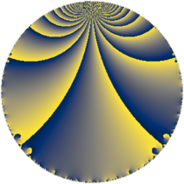# Properties

 Label 48.3.lLevel $48$ Weight $3$ Character orbit 48.l Rep. character $\chi_{48}(19,\cdot)$ Character field $\Q(\zeta_{4})$ Dimension $16$ Newform subspaces $1$ Sturm bound $24$ Trace bound $0$

# Related objects

## Defining parameters

 Level: $$N$$ $$=$$ $$48 = 2^{4} \cdot 3$$ Weight: $$k$$ $$=$$ $$3$$ Character orbit: $$[\chi]$$ $$=$$ 48.l (of order $$4$$ and degree $$2$$) Character conductor: $$\operatorname{cond}(\chi)$$ $$=$$ $$16$$ Character field: $$\Q(i)$$ Newform subspaces: $$1$$ Sturm bound: $$24$$ Trace bound: $$0$$

## Dimensions

The following table gives the dimensions of various subspaces of $$M_{3}(48, [\chi])$$.

Total New Old
Modular forms 36 16 20
Cusp forms 28 16 12
Eisenstein series 8 0 8

## Trace form

 $$16 q + 12 q^{4} - 12 q^{8} + O(q^{10})$$ $$16 q + 12 q^{4} - 12 q^{8} - 56 q^{10} + 32 q^{11} - 24 q^{12} - 44 q^{14} + 32 q^{16} + 12 q^{18} - 32 q^{19} + 80 q^{20} + 32 q^{22} - 128 q^{23} + 36 q^{24} - 100 q^{26} - 120 q^{28} + 32 q^{29} + 72 q^{30} + 160 q^{32} + 96 q^{34} + 96 q^{35} + 12 q^{36} - 96 q^{37} + 168 q^{38} + 48 q^{40} - 60 q^{42} + 160 q^{43} + 88 q^{44} + 136 q^{46} - 144 q^{48} + 112 q^{49} - 236 q^{50} - 96 q^{51} - 48 q^{52} - 160 q^{53} - 36 q^{54} - 256 q^{55} - 224 q^{56} + 144 q^{58} - 128 q^{59} - 72 q^{60} - 32 q^{61} - 276 q^{62} - 408 q^{64} - 32 q^{65} + 72 q^{66} + 320 q^{67} - 448 q^{68} + 96 q^{69} - 384 q^{70} + 512 q^{71} + 60 q^{72} + 348 q^{74} + 192 q^{75} + 72 q^{76} + 224 q^{77} + 396 q^{78} + 552 q^{80} - 144 q^{81} - 40 q^{82} - 160 q^{83} + 72 q^{84} + 160 q^{85} + 528 q^{86} + 480 q^{88} - 24 q^{90} - 480 q^{91} + 496 q^{92} + 312 q^{94} - 480 q^{96} - 440 q^{98} + 96 q^{99} + O(q^{100})$$

## Decomposition of $$S_{3}^{\mathrm{new}}(48, [\chi])$$ into newform subspaces

Label Dim $A$ Field CM Traces $q$-expansion
$a_{2}$ $a_{3}$ $a_{5}$ $a_{7}$
48.3.l.a $16$ $1.308$ $$\mathbb{Q}[x]/(x^{16} - \cdots)$$ None $$0$$ $$0$$ $$0$$ $$0$$ $$q-\beta _{3}q^{2}-\beta _{5}q^{3}+(1-\beta _{2})q^{4}+(\beta _{1}+\cdots)q^{5}+\cdots$$

## Decomposition of $$S_{3}^{\mathrm{old}}(48, [\chi])$$ into lower level spaces

$$S_{3}^{\mathrm{old}}(48, [\chi]) \cong$$ $$S_{3}^{\mathrm{new}}(16, [\chi])$$$$^{\oplus 2}$$